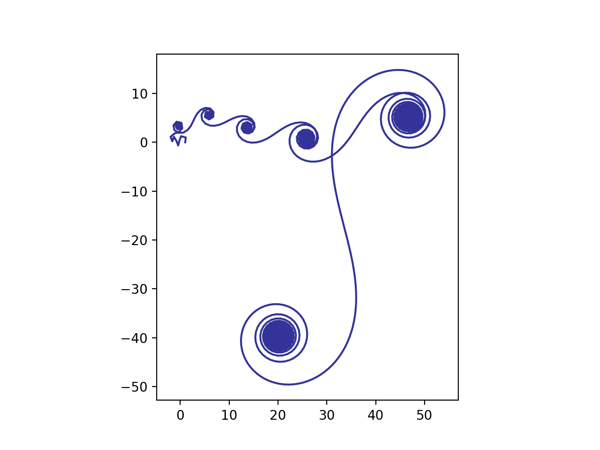# Exponential sums make interesting pictures

Exponential sums are a specialized area of math that studies series with terms that are complex exponentials.

$$\sum\limits_{n=1}^{N}{e{}^{2\pi if(n)}}$$

Exponential sums also make pretty pictures. If you make a scatter plot of the sequence of partial sums you can get surprising shapes. This is related to the trickiness of estimating such sums: the partial sums don’t simply monotonically converge to a limit. By the plot of an exponential sum we mean the sequence of partial sums, plotted in the complex plane, with successive points joined by straight line segments. That is, we start at the origin; draw a line interval corresponding to the first term of the sum; from the end of this interval draw another, corresponding to the second term of the sum; and so on.

Following this article, we playing around with polynomials with dates in the denominator. If we take that suggestion, with

$$f(n)=\frac{n}{dd}+\frac{{n}^{2}}{mm}+\frac{{n}^{3}}{yy}$$

and with today’s date, we get the curve below:Here’s the python code that produced the image.
import matplotlib.pyplot as plt
from numpy import array, pi, exp, log
N = 20000
def f(n):
return n/25 + n**2/11+ n**3/20
z = array( [exp( 2*pi*1j*f(n) ) for n in range(0, N)] )
z = z.cumsum()

plt.plot(z.real, z.imag, color='#333399')
plt.axes().set_aspect(1)
plt.show()

An interesting picture is the exponential sum with f(n)=(log n)4 and N=5000. The graph was dubbed “the Loch Ness monster” by John Loxton in a 1981 article.